User’s Guide : Multiple Equation Analysis : Models : Using Add Factors

Normally, when a model is solved deterministically, the equations of the model are solved so that each of the equations of the model is exactly satisfied. When a model is solved stochastically, random errors are added to each equation, but the random errors are still chosen so that their average value is zero.
If we have no information as to the errors in our stochastic equations that are likely to occur during the forecast period, then this behavior is appropriate. If, however, we have additional information as to the sort of errors that are likely during our forecast period, then we may incorporate that information into the model using add factors.
The most common use for add factors is to provide a smoother transition from historical data into the forecast period. Typically, add factors will be used to compensate for a poor fit of one or more equations of the model near the end of the historical data, when we suspect this will persist into the forecast period. Add factors provide an ad hoc way of trying to adjust the results of the model without respecifying or reestimating the equations of the model.
In reality, an add factor is just an extra exogenous variable which is included in the selected equation in a particular way. EViews allows an add factor to take one of two forms. If our equation has the form: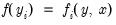(51.3)
then we can provide an add factor for the equation intercept or residual by simply including the add factor at the end of the equation: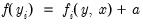(51.4)
Alternatively, we may provide an add factor for the endogenous variable of the model by using the add factor as an offset: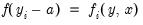(51.5)
where the sign of the add factor is reversed so that it acts in the same direction as for the previous case.
If the endogenous variable appears by itself on the left hand side of the equal sign, then the two types of add factor are equivalent. If the endogenous variable is contained in an expression, for example, a log transformation, then this is no longer the case. Although the two add factors will have a similar effect, they will be expressed in different units with the former in the units of the residual of the equation, and the latter in the units of the endogenous variable of the equation.
There are two ways to include add factors. The easiest way is to go to the equation view of the model, then double click on the equation in which you would like to include an add factor.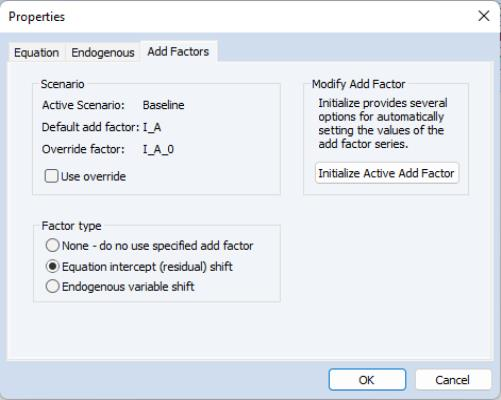When the equation properties dialog appears, switch to the Add Factors tab. In the Factor type box, select whether you would like an intercept or an endogenous variable shift add factor. A message box will prompt for whether you would like to create a series in the workfile to hold the add factor values. Click on Yes to create the series.
The series will initially be filled with NAs. You can initialize the add factor using one of several methods by clicking on the Initialize Active Add Factor button.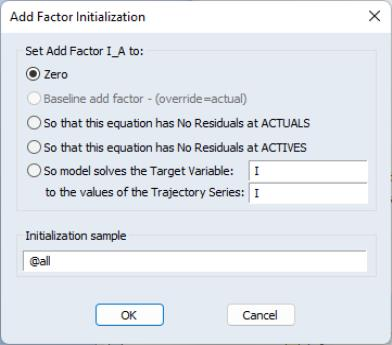A dialog box will come up offering the following options:
Zero: set the add factor to zero for every period.
So that this equation has no residuals at actuals: set the values of the add factor so that the equation is exactly satisfied without error when the variables of the model are set to the values contained in the actual series (typically the historical data).
So that this equation has no residuals at actives: set the values of the add factor so that the equation is exactly satisfied without error when the variables of the model are set to the values contained in the endogenous and exogenous series associated with the active scenario.
So model solves the target variable to the values of the trajectory series: set the values of the add factor so that an endogenous variable of the model follows a particular target path when the model is solved.
You can also change the sample over which you would like the add factor to be initialized by modifying the Initialization sample box. Click on OK to accept the settings.
Once an add factor has been added to an equation, it will appear in the variable view of the model as an additional variable. If an add factor is present in any scenario, then it must be present in every scenario, although the values of the add factor can be overridden for a particular scenario in the same way as for an exogenous variable.
The second way to handle add factors is to assign, initialize or override them for all the equations in the model at the same time using the Proc/Add Factors menu from the model window. For example, to create a complete set of add factors that make the model solve to actual values over history, we can use Add Factors/Equation Assignment... to create add factors for every equation, then use Add Factors/Set Values... to set the add factors so that all the equations have no residuals at the actual values.
When solving a model with an add factor, any missing values in the add factor will be treated as zeros.